# Characteristic Lengths in Superconductors

Arising in the theoretical and experimental investigations of superconductivity are two characteristic lengths, the London penetration depth and the coherence length.

The London penetration depth refers to the exponentially decaying magnetic field at the interior surface of a superconductor. It is related to the density of superconducting electrons in the material. The fact of exclusion of magnetic fields from the interior of the superconductor is called the Meissner effect.

An independent characteristic length is called the coherence length. It is related to the Fermi velocity for the material and the energy gap associated with the condensation to the superconducting state. It has to do with the fact that the superconducting electron density cannot change quickly - there is a minimum length over which a given change can be made, lest it destroy the superconducting state. For example, a transition from the superconducting state to a normal state will have a transition layer of finite thickness which is related to the coherence length. Experimental studies of various superconductors have led to the following calculated values for these two types of characteristic lengths.

 Material Coherence length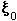(nm) London penetration depth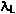(nm) Ratio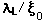Sn 230 34 0.16 Al 1600 16 0.010 Pb 83 37 0.45 Cd 760 110 0.14 Nb 38 39 1.02
Data attributed to R. Meservey and B. B. Schwartz.
Index

Superconductivity concepts

Reference
Kittel,
Ch 12

 HyperPhysics***** Condensed Matter R Nave
Go Back

# London Penetration Depth in SuperconductorsOne of the theoretical approches to the description of the superconducting state is the London equation. It relates the curl of the current density J to the magnetic field: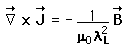The London equation can be shown to require that the magnetic field exponentially decays to zero inside a superconductor (see Meissner effect discussion). The nature of the decay (see Chapter 12 of Kittel) depends upon the superconducting electron density n:Characteristic lengths in superconductors
Index

Superconductivity concepts

Reference
Kittel,
Ch 12

 HyperPhysics***** Condensed Matter R Nave
Go Back

# Coherence Length in Superconductors

One of the characteristic lengths for the description of superconductors is called the coherence length. It is related to the Fermi velocity for the material and the energy gap associated with the condensation to the superconducting state. It has to do with the fact that the superconducting electron density cannot change quickly - there is a minimum length over which a given change can be made, lest it destroy the superconducting state. For example, a transition from the superconducting state to a normal state will have a transition layer of finite thickness which is related to the coherence length.Index

Superconductivity concepts

Reference
Kittel,
Ch 12

 HyperPhysics***** Condensed Matter R Nave
Go Back

# Meissner Effect from London Equation

The London equation relates the curl of the current density J to the magnetic field:By relating the London equation to Maxwell's equations, it can be shown that the Meissner effect arises from the London equation. One of Maxwell's equations is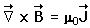Using the vector calculus identity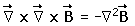along with the curl of the Maxwell equation above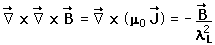and by substitution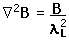Since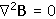by Maxwell's equations, the value for B inside the superconductor must be identically zero unless the penetration depth is infinite (i.e., not a superconductor). This is one of the theoretical approaches to explaining the Meissner effect.

Index

Superconductivity concepts

Reference
Kittel,
Ch 12

 HyperPhysics***** Condensed Matter R Nave
Go Back